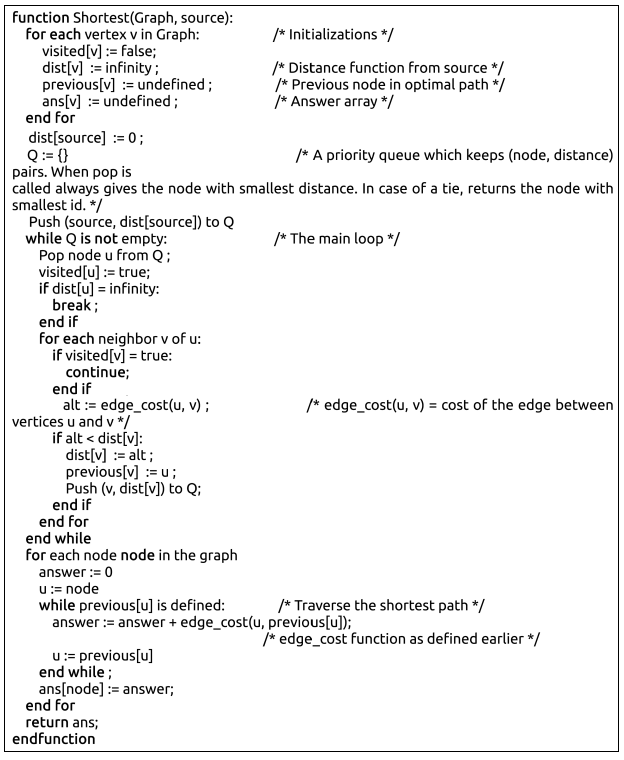# UVa 12717 - Fiasco

## Problem

Natasha always mixes up things in her algorithm classes. Last week Prof. Chhaya Murthy gave the class a classical problem -finding shortest path to all nodes from a special node of a weighted graph. Before that in another class, the professor taught them Prim’s and Dijkstra’s algorithms which are similar looking but does very different things. Always confused, poor Natasha submitted something
very similar to the Prim’s algorithm (actually we should call it Natasha’s lgorithm), she didn’t test it properly. Pseudocode of her algorithm looks as followsAfter submission, she showed the code to a friend who is good at algorithms. That friend immediately found the aw. Natasha was really terrifed and started to cry. Then there came the guys, the guys who liked her. They jumped at the opportunity and got hold of the dataset to be used to test the solutions. Your friend Rehan is one of those guys. He really wanted to impress Natasha. He asked you to change the dataset in such a way that Natasha’s algorithm gives the right result. After the change, Rehan will somehow be able to put the modified data with the old timestamp. Rehan told you that:

1. The dataset has T test cases.
2. Each case contains a connected weighted undirected graph with n nodes and
m edges.
3. Each case will contain a source node source.
4. Edge weights are positive and distinct.

To avoid suspicion, Rehan asked you not to change which vertices the edges connect, or the overall set of edge weights. You may only reorder which weights are assigned to which edges

## Input

The first line of the input denotes the number of datasets T (1 < T <= 15). T sets of case will follow. Each case will start with a triplet of numbers n (2 <= n <= 2500), m (1 <= m <= 25000) and source (1 <= source <= n) | the number of nodes, the number of edges and the starting node respectively.

Each of the next m lines will contain a triplet of numbers `(u, v, w)` meaning that there is an edge between node u and node v with weight w (1 <= w <= m). Nodes are numbered from 1 to n.

It is guaranteed that there is no duplicate or self-edges in the input and the graph is connected. In each given graph, all edge weights will be distinct.

## Output

For each set of input, print one set of output. First line of a set should be of the format, `Case X:` where X is the case number. Then print each edge triplet | one triplet (u, v, w) in each line separated by a single space. The edges should be printed in the order given in the input. If there is more than one solution to a dataset, any one of them will do.

## Solution

// 給一個錯誤代碼，修改測資，使錯誤代碼輸出正確。